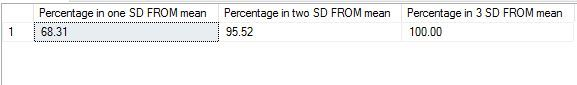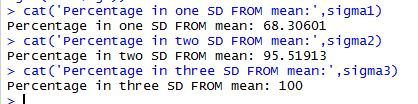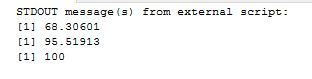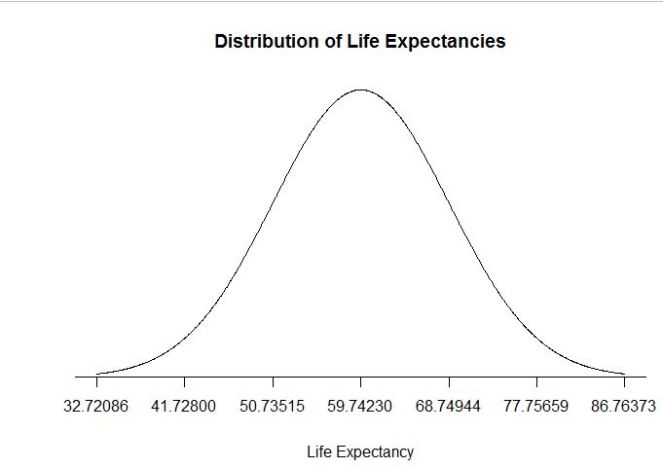# The Empirical Rule

, 2017-03-02 (first published: )

I am resuming technical blogging after a gap of nearly a month. I will continue to blog my re learning of statistics and basic concepts, and illustrate them to the best of my ability using R and T-SQL where appropriate.

For this week I have chosen a statistical concept called ‘Empirical Rule’.

The empirical rule is a test to determine if or not the dataset belongs to a normal distribution.

• 68% of data falls within the first standard deviation from the mean.
• 95% fall within two standard deviations.
• 99.7% fall within three standard deviations.

The rule is also called the 68-95-99 7 Rule or the Three Sigma Rule. The basic reason to test if a dataset follows this rule is to determine whether or not it is a normal distribution. When it is normally distributed and follows the bell curve – it lends itself easily to a variety of statistical analysis, especially forecasting. There are many other tests for normalisation that are more rigorous than this – and the wiki link has a good summary of those, but this is a basic and easy test.

For my test purpose I used the free dataset from WHO 0n life expectancies around the globe. It can be found here. I imported this dataset into sql server. I ran a simple TSQL script to find out if this dataset satisfies the empirical rule.

```DECLARE @sdev numeric(18,2), @mean numeric(18, 2), @sigma1 numeric(18, 2), @sigma2 numeric(18, 2), @sigma3 numeric(18, 2)
DECLARE @totalcount numeric(18, 2)
SELECT @sdev = SQRT(var(age)) FROM [dbo].[WHO_LifeExpectancy]
SELECT @mean = sum(age)/count(*) FROM [dbo].[WHO_LifeExpectancy]
SELECT @totalcount = count(*) FROM [dbo].[WHO_LifeExpectancy]
SELECT @sigma1 = (count(*)/@totalcount)*100 FROM [dbo].[WHO_LifeExpectancy] WHERE age >= @mean-@sdev and age<= @mean+@sdev
SELECT @sigma2 = (count(*)/@totalcount)*100 FROM [dbo].[WHO_LifeExpectancy] WHERE age >= @mean-(2*@sdev) and age<= @mean+(2*@sdev)
SELECT @sigma3 = (count(*)/@totalcount)*100 FROM [dbo].[WHO_LifeExpectancy] WHERE age >= @mean-(3*@sdev) and age<= @mean+(3*@sdev)
SELECT @sigma1 AS 'Percentage in one SD FROM mean', @sigma2 AS 'Percentage in two SD FROM mean',
@sigma3 as 'Percentage in 3 SD FROM mean'```

The results I got are as below. As we can see ,68.31 % of data falls between one difference of mean and standard deviation, 95 % falls between two differences and 100% falls between 3. This is a near perfect normal distribution – one that lends itself to statistical analysis very easily.To do the same analysis in R, I used script as below:

```install.packages("RODBC")
library(RODBC)
cn <- odbcDriverConnect(connection="Driver={SQL Server Native Client 11.0};server=MALATH-PC\\SQL01;database=WorldHealth;Uid=sa;Pwd=Mysql1")
data <- sqlQuery(cn, 'select age from [dbo].[WHO_LifeExpectancy] where age is not null')
agemean<-mean(data\$age)
agesd<-sd(data\$age)
sigma1<-(sum(data\$age>=agemean-agesd & data\$age<=agemean+agesd)/length(data\$age))*100
sigma2<-(sum(data\$age>=agemean-(2*agesd) & data\$age<=agemean+(2*agesd))/length(data\$age))*100
sigma3<-(sum(data\$age>=agemean-(3*agesd) & data\$age<=agemean+(3*agesd))/length(data\$age))*100
cat('Percentage in one SD FROM mean:',sigma1)
cat('Percentage in two SD FROM mean:',sigma2)
cat('Percentage in three SD FROM mean:',sigma3)```

My results are as below:I can use the same script within TSQL and call it –

```EXEC sp_execute_external_script
@language = N'R'
,@script = N'agemean<-mean(InputDataSet\$age)
agesd<-sd(InputDataSet\$age)
sigma1<-(sum(InputDataSet\$age>=agemean-agesd & InputDataSet\$age<=agemean+agesd)/length(InputDataSet\$age))*100
sigma2<-(sum(InputDataSet\$age>=agemean-(2*agesd) & InputDataSet\$age<=agemean+(2*agesd))/length(InputDataSet\$age))*100
sigma3<-(sum(InputDataSet\$age>=agemean-(3*agesd) & InputDataSet\$age<=agemean+(3*agesd))/length(InputDataSet\$age))*100
print(sigma1)
print(sigma2)
print(sigma3)'
,@input_data_1 = N'select age from [dbo].[WHO_LifeExpectancy] where age is not null;'```As we can see, the results are exactly the same in all 3 methods. We can also draw a bell curve with this data in R and see that it is a perfect curve, proving that the data is from a normally distributed set. The code I wrote to draw the graph is as below:

```lower_bound <- agemean - agesd * 3
upper_bound <- agemean + agesd * 3
x <- seq(-3, 3, length = 1000) * agesd + agemean
y <- dnorm(x, agemean, agesd)
plot(x, y, type="n", xlab = "Life Expectancy", ylab = "", main = "Distribution of Life Expectancies", axes = FALSE)
lines(x, y)
sd_axis_bounds = 5
axis_bounds <- seq(-sd_axis_bounds * agesd + agemean, sd_axis_bounds * agesd + agemean, by = agesd)
axis(side = 1, at = axis_bounds, pos = 0)```# Book Review: Big Red - Voyage of a Trident Submarine

I've grown up reading Tom Clancy and probably most of you have at least seen Red October, so this book caught my eye when browsing used books for a recent trip. It's a fairly human look at what's involved in sailing on a Trident missile submarine...

2009-03-10

1,439 reads

# Database Mirroring FAQ: Can a 2008 SQL instance be used as the witness for a 2005 database mirroring setup?

Question: Can a 2008 SQL instance be used as the witness for a 2005 database mirroring setup? This question was sent to me via email. My reply follows. Can a 2008 SQL instance be used as the witness for a 2005 database mirroring setup? Databases to be mirrored are currently running on 2005 SQL instances but will be upgraded to 2008 SQL in the near future.

2009-02-23

1,567 reads

# Inserting Markup into a String with SQL

In which Phil illustrates an old trick using STUFF to intert a number of substrings from a table into a string, and explains why the technique might speed up your code...

2009-02-18

1,631 reads

# Networking - Part 4

You may want to read Part 1 , Part 2 , and Part 3 before continuing. This time around I'd like to talk about social networking. We'll start with social networking. Facebook, MySpace, and Twitter are all good examples of using technology to let...

2009-02-17

1,530 reads

# Speaking at Community Events - More Thoughts

Last week I posted Speaking at Community Events - Time to Raise the Bar?, a first cut at talking about to what degree we should require experience for speakers at events like SQLSaturday as well as when it might be appropriate to add additional focus/limitations on the presentations that are accepted. I've got a few more thoughts on the topic this week, and I look forward to your comments.

2009-02-13

360 reads